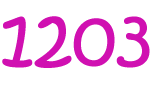Pull Down & Release to Refresh

# Definition of QuaternaryQuaternary numbers are made up only of the digits $\{0,1,2,3\}$. Each place in a quaternary number corresponds to a power of $4$.

Because there are only $4$ different possible digits in a quaternary number, we also call them Base $4$ numbers.

For example, the quaternary number $1203$ is equal to the decimal number $1 \times 4^3 + 2 \times 4^2 + 0 \times 4^1 + 3 \times 4^0 = 64 + 2 \times 16 + 0 + 3 = 99$.

Some other examples of quaternary numbers are:

• $33$, which corresponds to the decimal number $15$.
• $300$, which corresponds to the decimal number $48$.
• $101$, which corresponds to the decimal number $17$.

### Description

The aim of this dictionary is to provide definitions to common mathematical terms. Students learn a new math skill every week at school, sometimes just before they start a new skill, if they want to look at what a specific term means, this is where this dictionary will become handy and a go-to guide for a student.

### Audience

Year 1 to Year 12 students

### Learning Objectives

Learn common math terms starting with letter Q

Author: Subject Coach
You must be logged in as Student to ask a Question.